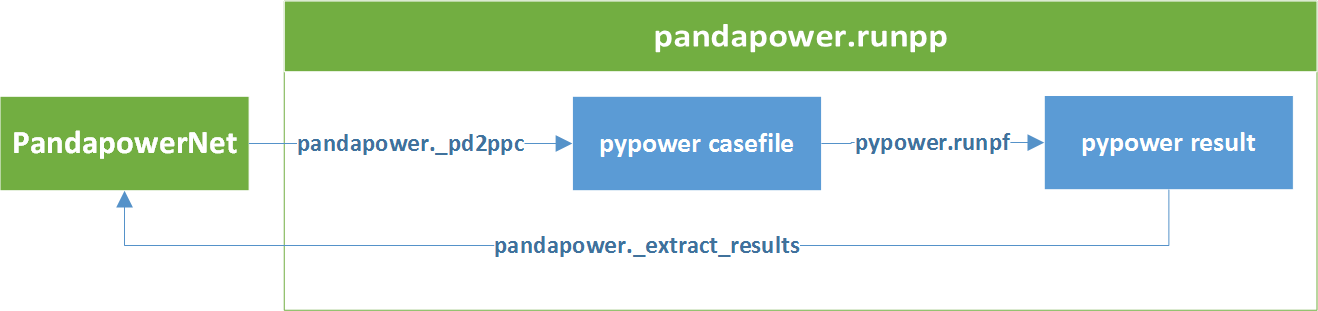# Power Flow¶

pandapower uses PYPOWER to solve the power flow problem:pandapower.runpp(net, algorithm='nr', calculate_voltage_angles='auto', init='auto', max_iteration='auto', tolerance_kva=1e-05, trafo_model='t', trafo_loading='current', enforce_q_lims=False, numba=True, recycle=None, check_connectivity=True, r_switch=0.0, voltage_depend_loads=True, delta_q=0, **kwargs)

Runs PANDAPOWER AC Flow

INPUT:
net - The pandapower format network
OPTIONAL:

algorithm (str, “nr”) - algorithm that is used to solve the power flow problem.

The following algorithms are available:

• “nr” newton-raphson (pypower implementation with numba accelerations)
• “bfsw” backward/forward sweep (specially suited for radial and weakly-meshed networks)
• “gs” gauss-seidel (pypower implementation)
• “fdbx” (pypower implementation)
• “fdxb”(pypower implementation)

calculate_voltage_angles (bool, “auto”) - consider voltage angles in loadflow calculation

If True, voltage angles of ext_grids and transformer shifts are considered in the loadflow calculation. Considering the voltage angles is only necessary in meshed networks that are usually found in higher networks. Thats why calculate_voltage_angles in “auto” mode defaults to:

• True, if the network voltage level is above 70 kV
• False otherwise

The network voltage level is defined as the maximum rated voltage in the network that is connected to a line.

init (str, “auto”) - initialization method of the loadflow pandapower supports four methods for initializing the loadflow:

• “auto” - init defaults to “dc” if calculate_voltage_angles is True or “flat” otherwise
• “flat”- flat start with voltage of 1.0pu and angle of 0° at all PQ-buses and 0° for PV buses as initial solution
• “dc” - initial DC loadflow before the AC loadflow. The results of the DC loadflow are used as initial solution for the AC loadflow.
• “results” - voltage vector of last loadflow from net.res_bus is used as initial solution. This can be useful to accelerate convergence in iterative loadflows like time series calculations.

Considering the voltage angles might lead to non-convergence of the power flow in flat start. That is why in “auto” mode, init defaults to “dc” if calculate_voltage_angles is True or “flat” otherwise

max_iteration (int, “auto”) - maximum number of iterations carried out in the power flow algorithm.

In “auto” mode, the default value depends on the power flow solver:

• 10 for “nr”
• 100 for “bfsw”
• 1000 for “gs”
• 30 for “fdbx”
• 30 for “fdxb”

tolerance_kva (float, 1e-5) - loadflow termination condition referring to P / Q mismatch of node power in kva

trafo_model (str, “t”) - transformer equivalent circuit model pandapower provides two equivalent circuit models for the transformer:

• “t” - transformer is modeled as equivalent with the T-model.
• “pi” - transformer is modeled as equivalent PI-model. This is not recommended, since it is less exact than the T-model. It is only recommended for valdiation with other software that uses the pi-model.

trafo_loading (str, “current”) - mode of calculation for transformer loading

Transformer loading can be calculated relative to the rated current or the rated power. In both cases the overall transformer loading is defined as the maximum loading on the two sides of the transformer.

• “current”- transformer loading is given as ratio of current flow and rated current of the transformer. This is the recommended setting, since thermal as well as magnetic effects in the transformer depend on the current.
• “power” - transformer loading is given as ratio of apparent power flow to the rated apparent power of the transformer.

enforce_q_lims (bool, False) - respect generator reactive power limits

If True, the reactive power limits in net.gen.max_q_kvar/min_q_kvar are respected in the loadflow. This is done by running a second loadflow if reactive power limits are violated at any generator, so that the runtime for the loadflow will increase if reactive power has to be curtailed.

Note: enforce_q_lims only works if algorithm=”nr”!

numba (bool, True) - Activation of numba JIT compiler in the newton solver

If set to True, the numba JIT compiler is used to generate matrices for the powerflow, which leads to significant speed improvements.

recycle (dict, none) - Reuse of internal powerflow variables for time series calculation

Contains a dict with the following parameters: _is_elements: If True in service elements are not filtered again and are taken from the last result in net[“_is_elements”] ppc: If True the ppc is taken from net[“_ppc”] and gets updated instead of reconstructed entirely Ybus: If True the admittance matrix (Ybus, Yf, Yt) is taken from ppc[“internal”] and not reconstructed

check_connectivity (bool, True) - Perform an extra connectivity test after the conversion from pandapower to PYPOWER

If True, an extra connectivity test based on SciPy Compressed Sparse Graph Routines is perfomed. If check finds unsupplied buses, they are set out of service in the ppc

r_switch (float, 0.0) - resistance of bus-bus-switches. If impedance is zero, buses connected by a closed bus-bus switch are fused to model an ideal bus. Otherwise, they are modelled as branches with resistance r_switch.

voltage_depend_loads (bool, True) - consideration of voltage-dependent loads. If False, net.load.const_z_percent and net.load.const_i_percent are not considered, i.e. net.load.p_kw and net.load.q_kvar are considered as constant-power loads.

delta_q - Reactive power tolerance for option “enforce_q_lims” in kvar - helps convergence in some cases.

**kwargs - options to use for PYPOWER.runpf

Warning

Neglecting voltage angles is only valid in radial networks! pandapower was developed for distribution networks, which is why omitting the voltage angles is the default. However be aware that voltage angle differences in networks with multiple galvanically coupled external grids lead to balancing power flows between slack nodes. That is why voltage angles always have to be considered in meshed network, such as in the sub-transmission level!

Note

If you are interested in the pypower casefile that pandapower is using for power flow, you can find it in net[“_ppc”]. However all necessary informations are written into the pandpower format net, so the pandapower user should not usually have to deal with pypower.